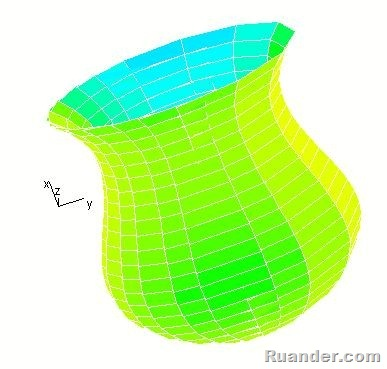## Pages

### Solids of RevolutionI was helping my brother the other day with an application for his math class. In this particular application they were asked to find the volume of a vase that is formed by rotating about the y-axis the region in the first quadrant bounded by the curves x=0.0437y3-0.7125y2+3.15y+4 and y=10. A solution for this problem can be implemented by creating a differential disk of radios x and thickness dy. The volume of the vase can then be found by integrating the differential volume of all disks from y=0 to y=10. Any way, to visualize the problem better, we plotted the resulting solid of revolution (shown in the picture) using Derive, which by the way it is a very useful program.